# Piecewise Linear Unit：分段线性激活函数

### 前言¶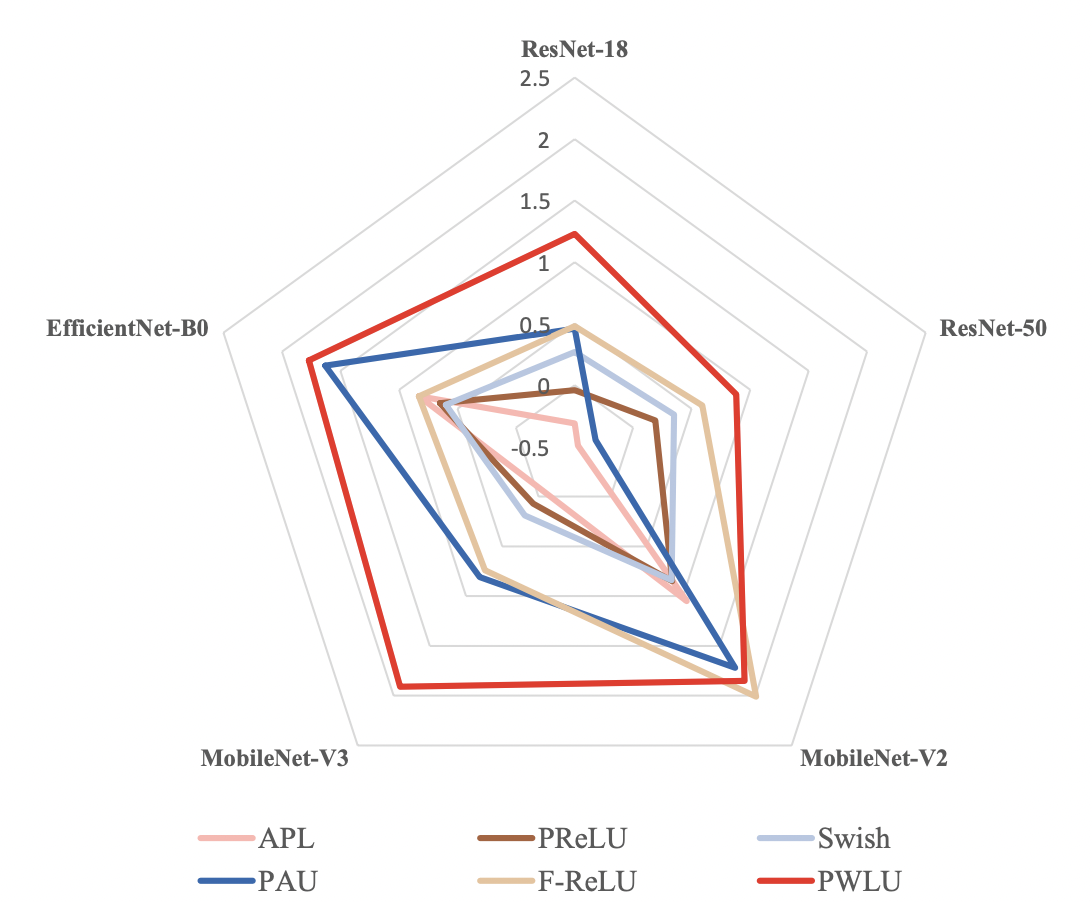### 方法¶

#### Piecewise Linear Unit的定义¶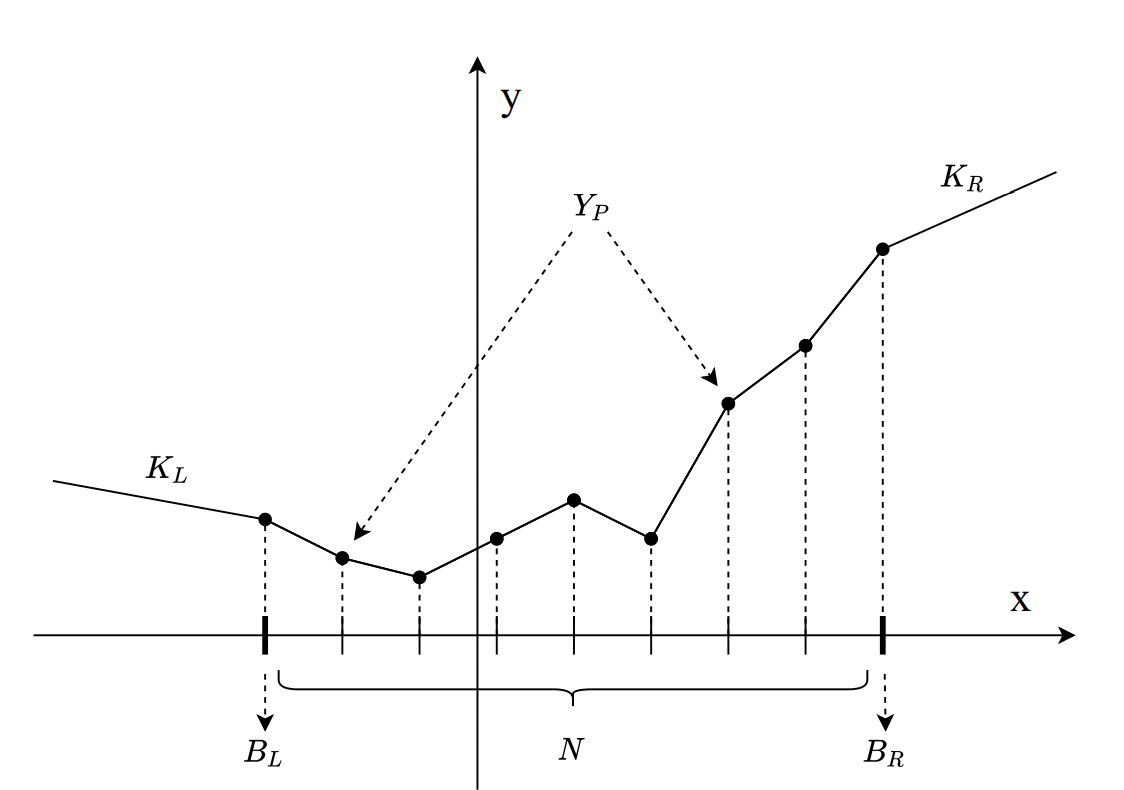• 分段数 N
• 左边界$B_L$，右边界$B_R$
• 每一段对应的Y轴值，$Y_P$
• 最左边界的斜率$K_L$，最右边界的斜率$K_R$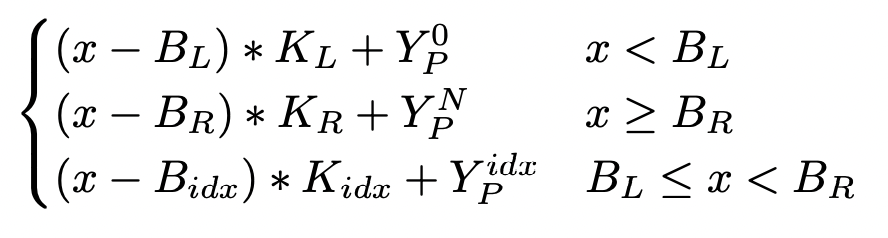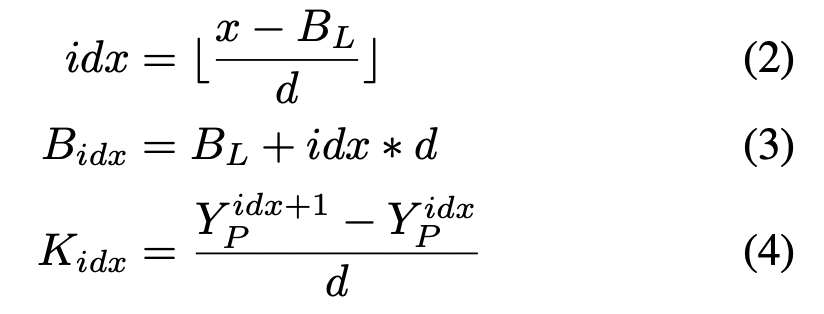• PWLU可以表示任意连续，有边界的scalar function
• PWLU变换连续，利于求导
• 可以最大限度利用可学习参数
• 由于我们划分段是N等分的，所以在计算，推理中是efficient的

#### Learning the Piecewise Linear Unit¶

• $B_R$ = -$B_L$
• $K_R$ = 1, $K_L$ = 0

##### 输入边界不对齐¶

PWLU中 $B_R$$B_L$ 是两个很重要的参数，他们限制了可学习的区域。显然，这个区域需要和输入的分布对齐。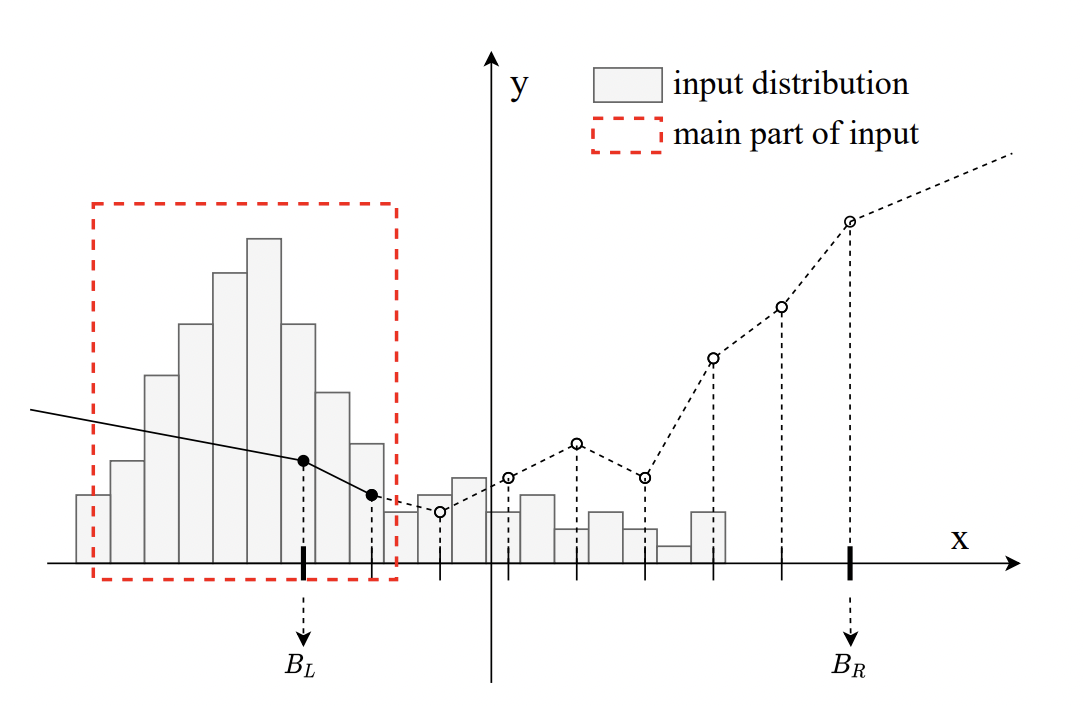• 阶段1: 在前面几轮，首先将PWLU设置为ReLU形式，并停止参数更新。计算移动平均得到的均值和方差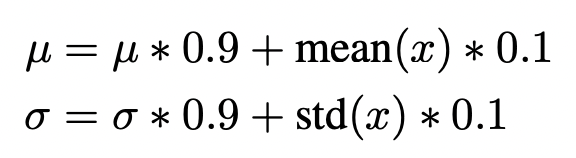• 阶段2: 开始PWLU的训练，应用3-sigma原则，设置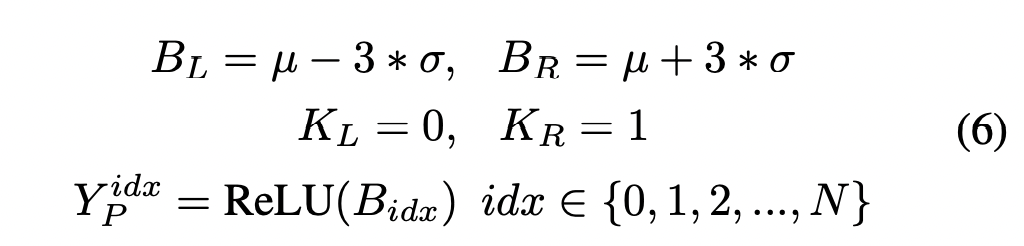### 实验结果¶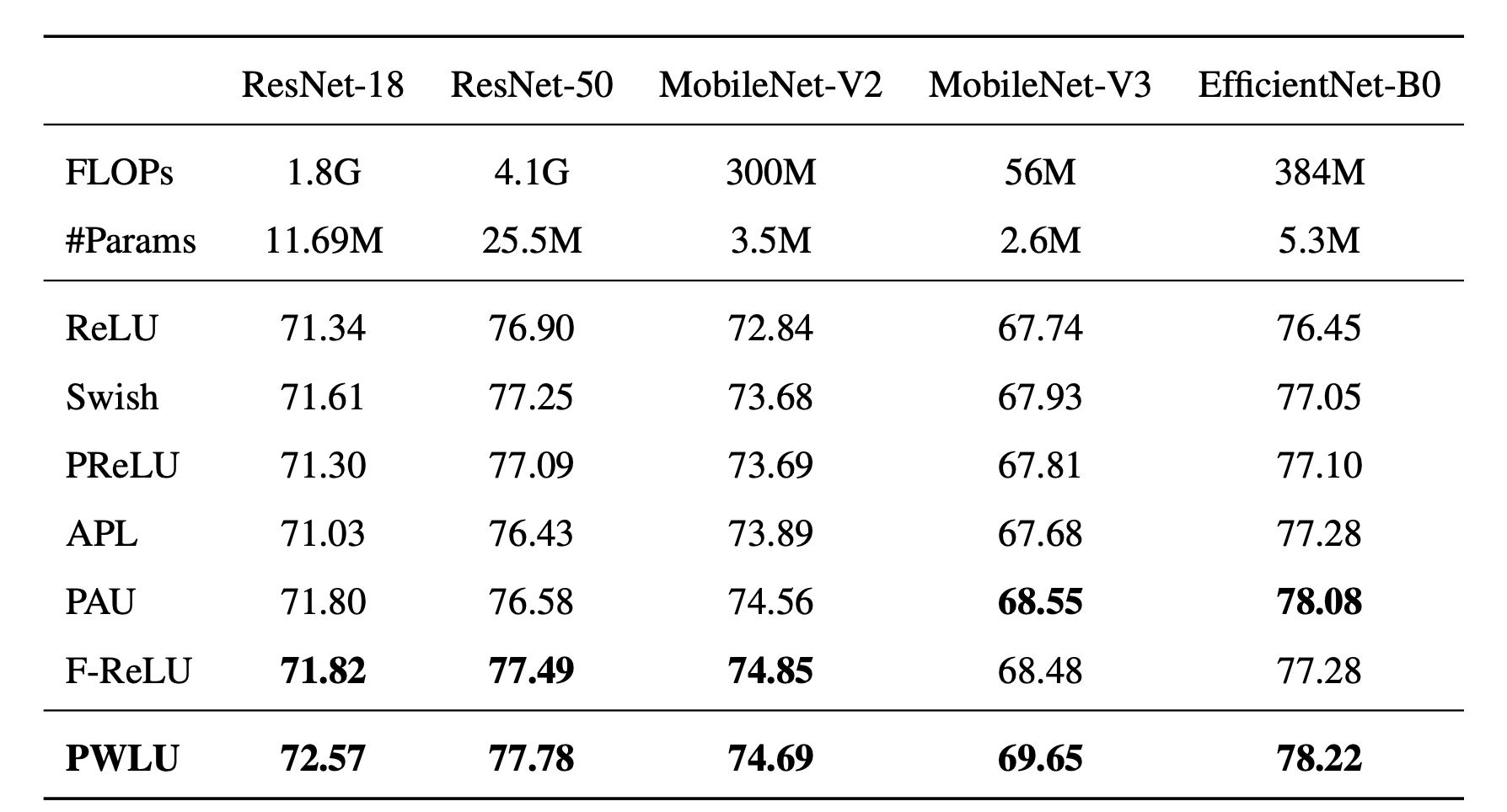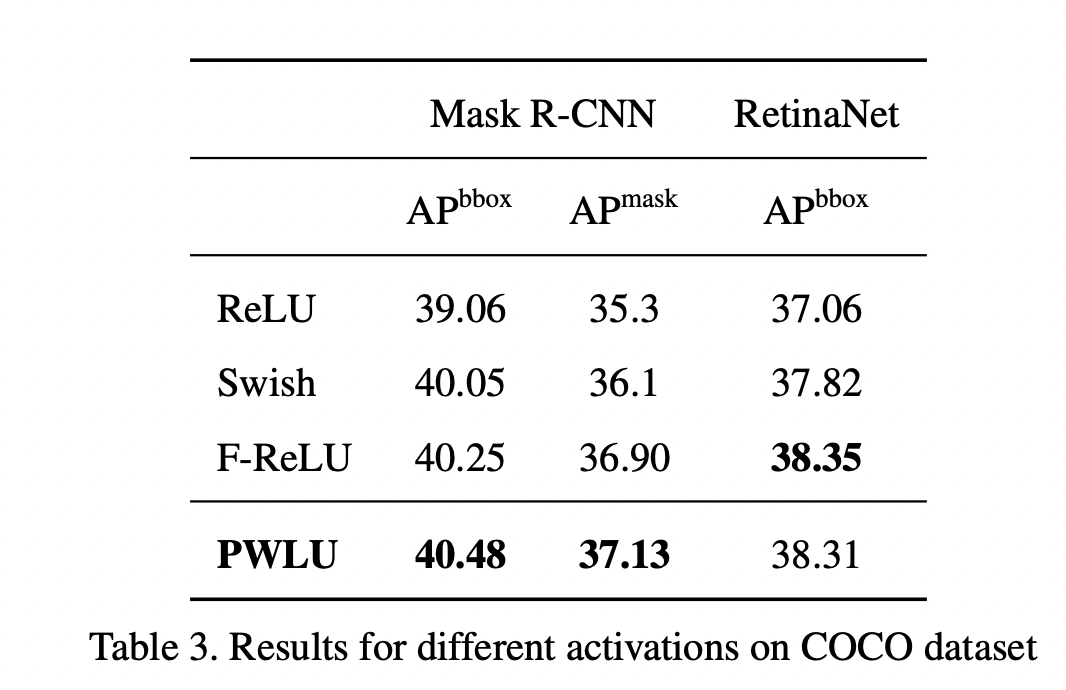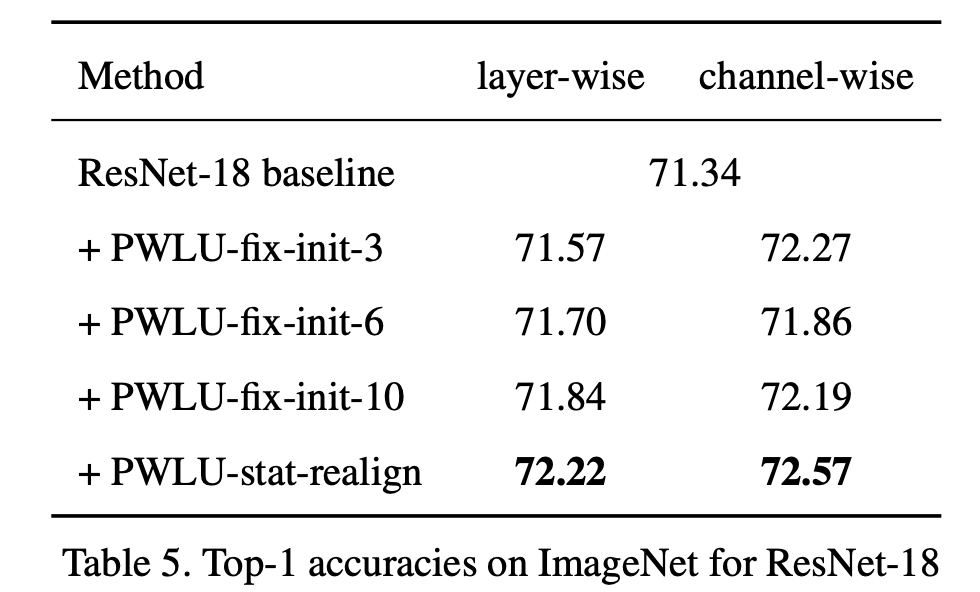### 可视化结果¶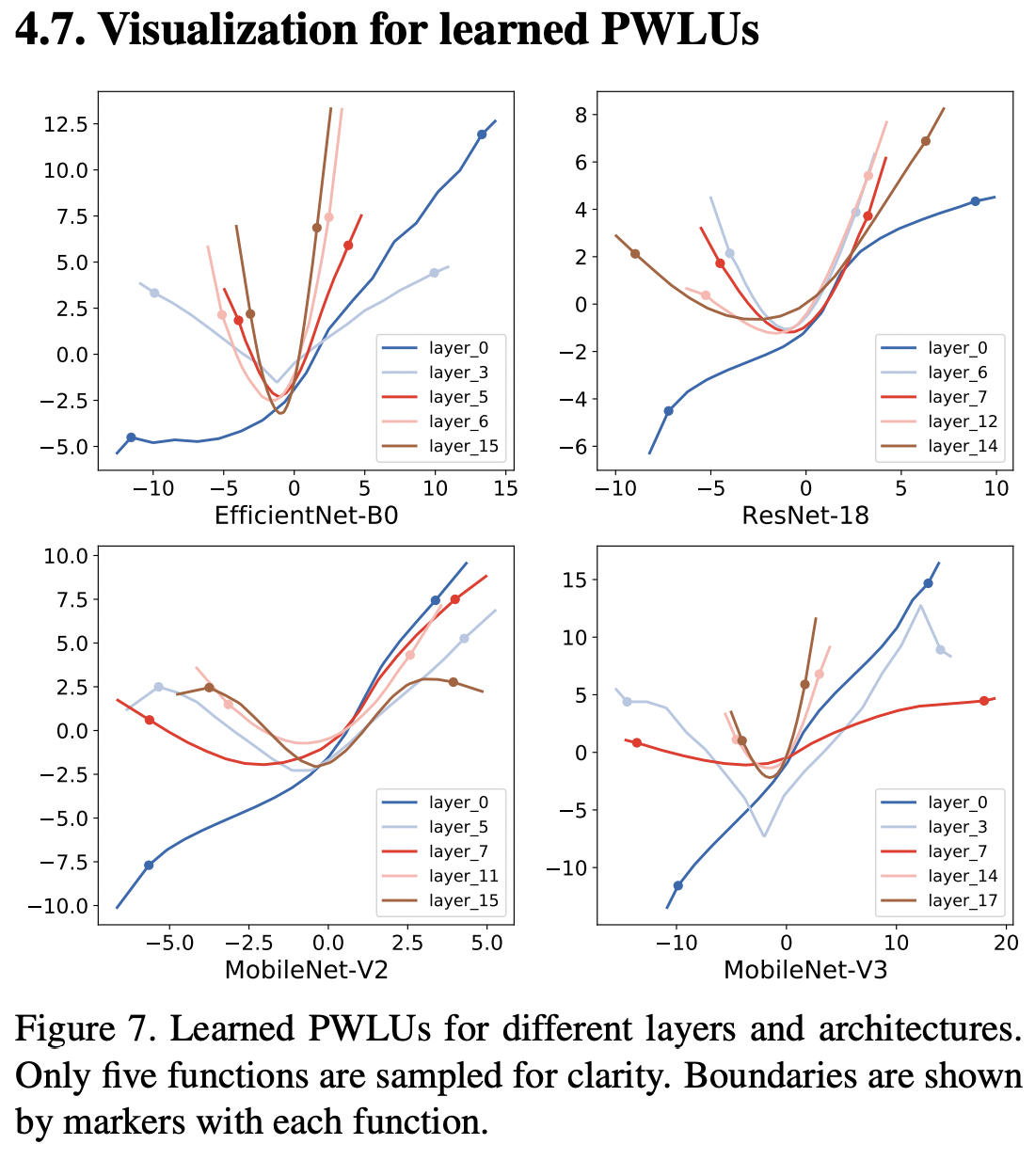### 非官方代码实现¶

github上有一个非官方代码实现，目前看来实现的有些错误，还不是很完善，仅供参考：

https://github.com/MrGoriay/pwlu-pytorch/blob/main/PWLA.py